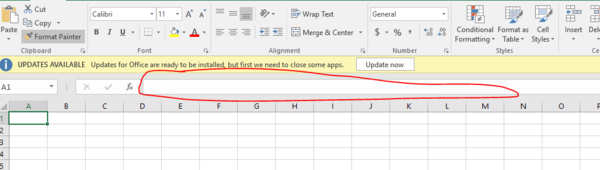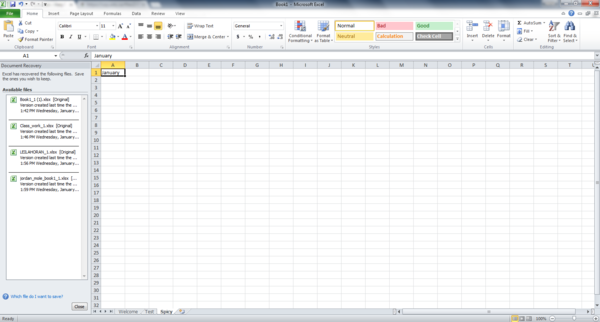##### Print Instructions

NOTE: Only your test content will print.
To preview this test, click on the File menu and select Print Preview.

See our guide on How To Change Browser Print Settings to customize headers and footers before printing.

# Introduction to Excel (Grade 8)

Print Test (Only the test content will print)

## Introduction to Excel

Instructions: The following questions will test your basic knowledge of Microsoft Excel. Good luck!

1.
The bar that shows the data/formula/phrase in the cell selected is the                             .
2.
The cell/table selected is also called the                                     .
3.
The cell that has been selected to input data is an                             .
4.
A rectangle box in a worksheet that contains data is a               .
5.
Formulas built into Excel are called                         .
6.
A set of instructions for Excel to carry out is a                     .
7.
An Excel file (with one or several worksheets) is a                       .
8.
A single spreadsheet within an Excel workbook is known as a                         .
9.
A group of cells running horizontally and represented by as number is a             .
10.
A series of cells which are numbered and run vertically.
11.
Which of the following does Microsoft Excel produce?
1. Worksheets
2. Tablets
3. Formulas
12.
Which of the following is the term for a single page or sheet in an Excel spreadsheet?
1. Workbook
2. Worksheet
3. Active Sheet
4. Formula
13.
Which of the following terms describes the item circled in the picture below?1. Auto Sum
2. Formula Bar
3. Equal sign
4. Name Box
14.
What is the name for the function in Microsoft Excel that automatically enters the appropriate formula or function into your spreadsheet?
1. Auto Sum
2. Auto Size
3. Auto Fill
4. Auto Mobile
15.
What is the name of sheet 3?1. Test
2. January
3. Spicy
4. Welcome
16.
What is missing in this formula? (A5+A7)/25
1. -
2. +
3. =
4. /
17.
Which of the following formulas is written correctly for Excel?
1. 3A+4A
2. =A3+A4
3. A3+4A
4. =A3+4A
18.
What is the term that describes the address or location of a cell (ex. B3 or C4)?